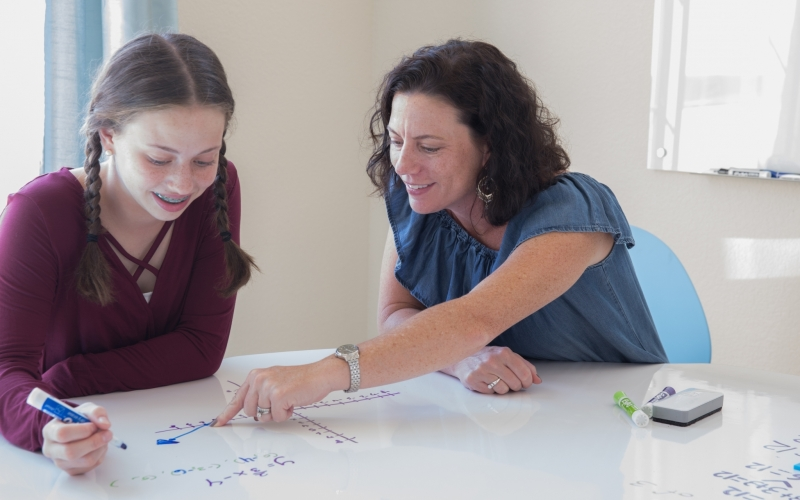Singapore Math Blog# What is the Singapore Math Curriculum?

Apr 25, 2021

The Singapore Math curriculum is a unique approach to mathematics education developed by the country’s Ministry of Education in 1982. Unlike regular Western mathematics, it focuses more on developing concepts and less on applied work. Since its inception, countries around the world have adopted it, using it as a tool to improve results.

## Incredible Results

The Singapore math approach uses the concrete-pictorial-abstract method - or CPA for short. The idea here is to adapt math education to a format that students find easier to understand. Instead of jumping in at the conceptual deep end, it attempts to construct a solid base first, and then build from there. Students don’t learn by rote memorization. Instead, they gain a conceptual understanding that allows them to solve problems better.

International studies support the effectiveness of the Singapore math approach. For instance, the results of the Trends in International Mathematics and Science study reveals that students that have undergone the Singapore math approach regularly outperform those who do not - including those on the US Common Core program. And this has captured the interest of parents in America and internationally.

At grade 4, for instance, 99 percent of students enrolled in Singapore math programs reach the international “low benchmark,” compared to 95 percent on regular curriculums. 80 percent reach the high benchmark on the Singapore math program, compared to just 47 on traditional curriculums. And just 14 percent of traditional math students achieve the advanced benchmark, compared to 50 percent on the Singapore program.

## The Method

Teachers of the Singapore math curriculum begin with concrete examples that express mathematical concepts in a tangible way and then progress from there. Thus, they begin with simple physical objects in the real world, such as oranges, and then build understanding between them and mathematical concepts. For instance, there might be four oranges in a bowl, and the teacher could ask the student to subtract two.

The next phase - the pictorial stage - switches out real objects for those drawn on a piece of paper. So instead of manipulating real objects with their hands, the child does so with images on the page. This stage still does not use full mathematical notation. But it shows students that you can represent math ideas without relying on the physical world.

The final step is the abstract one. This occurs when teachers represent mathematical concepts using symbols, such as numbers and operands (like plus, minus, multiply and divide).
This approach continues throughout the course. When explaining more abstract concepts, Singapore math textbooks will use bar charts and diagrams, thereby presenting ideas more intuitively.

## How can Singapore Math help my child?

If your child is struggling to learn math concepts, then online Singapore math could help. It complements the Common Core but provides a deeper understanding of critical concepts. This way, your child avoids learning topics by rote and, instead, learns them in a way they can apply to any problem.